Disclosure: This post may contain affiliate links, meaning when you click the links and make a purchase, we receive a commission.

# Excel formula to calculate hours worked and overtime [with template]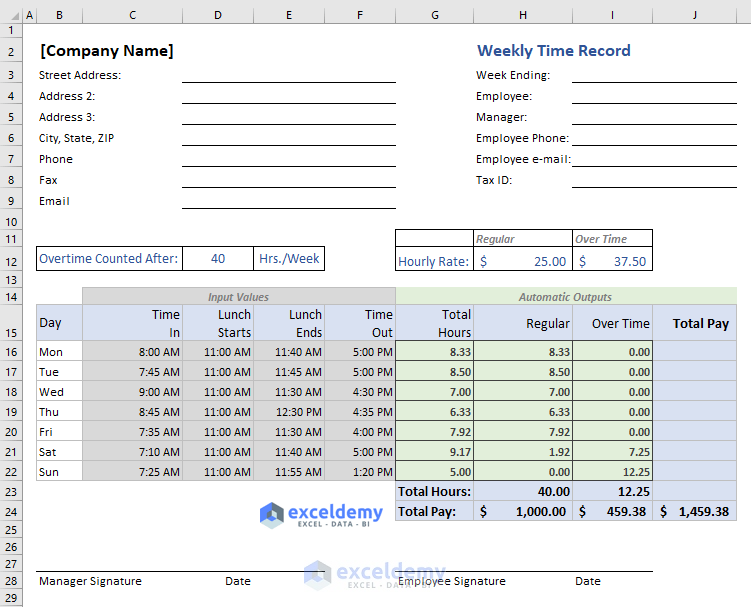It is easy to build an Excel formula to calculate hours worked and overtime of your employees. This is why Excel is widely used in the workplaces. It makes any calculation very easy.

Most companies calculate work hours and over time based on the 40-hours-work-week (8 hours per day). By default, my Excel template also calculate work hours and overtime based on the 40-hours-work-week. But if your company takes different hours-work-week, you can define that in the template.

In this Excel template, over time is calculated based on the whole week, not based on an individual day. Suppose, an employee has worked 9 hours on the very first day of the week. But his total hours in the week does not exceed 40 hours, he will not be rewarded any over time.

You can also define different hourly rates for Regular Works and Over Time Works.

## Excel formula to calculate hours worked and overtime

This is the template overview. This template is printable in one page (perfect for Letter and A4 Page with Landscape Orientation) without any modification.

### The inputs in the Excel Template

Put the following inputs in the Excel template (image below):Overtime Counted After: Generally, this is 40 hours per week. If your office maintains 45 or other working hours, input that value in this field. Working hours vary from country to country.

Hourly Rate:

Normally Working Hour Rate is different from the Over Time Hourly Rate.

• Regular: Input the Regular Hourly Rate
• Over Time: Input the Over Time Hourly Rate

The template takes 4 time-inputs (above image):

• Time In: This is the time when the employee enters into the working place.
• Lunch Starts: Time when the lunch starts in the working place.
• Lunch Ends: Lunch end time entry.
• Time Out: This is when the employee completely leaves the office place.

### Outputs we get automatically

These are the outputs we get from our inputs:

1) Total Hours:

This “Total Hours” is calculated for an individual day. We have used this formula in the cell G16 to get the total hours: =((F16-C16)-(E16-D16))*24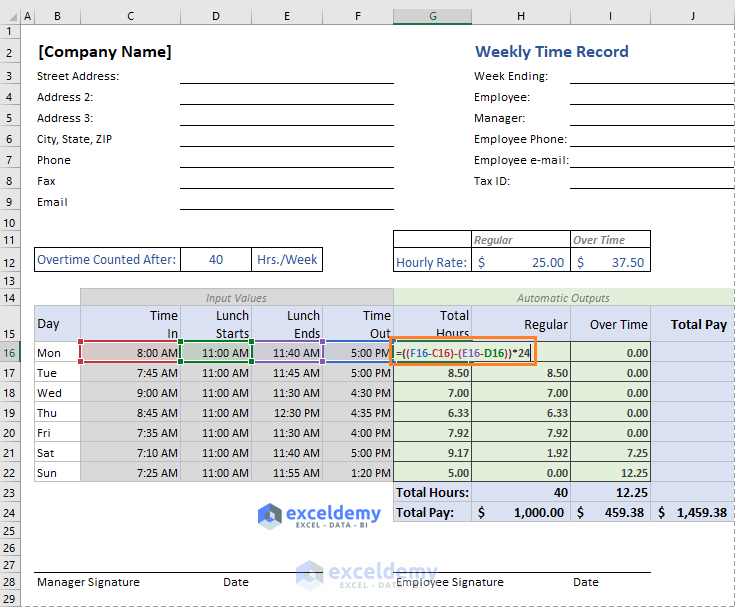• (F16-C16) is actually (Time Out – Time In). And (E16-D16) is (Lunch Ends – Lunch Starts).
• We multiplied ((Time Out – Time In) – (Lunch Ends – Lunch Starts)) by 24 to covert it into hour value.
• This is why we get the value in Number Otherwise, subtraction of two times results in Time format.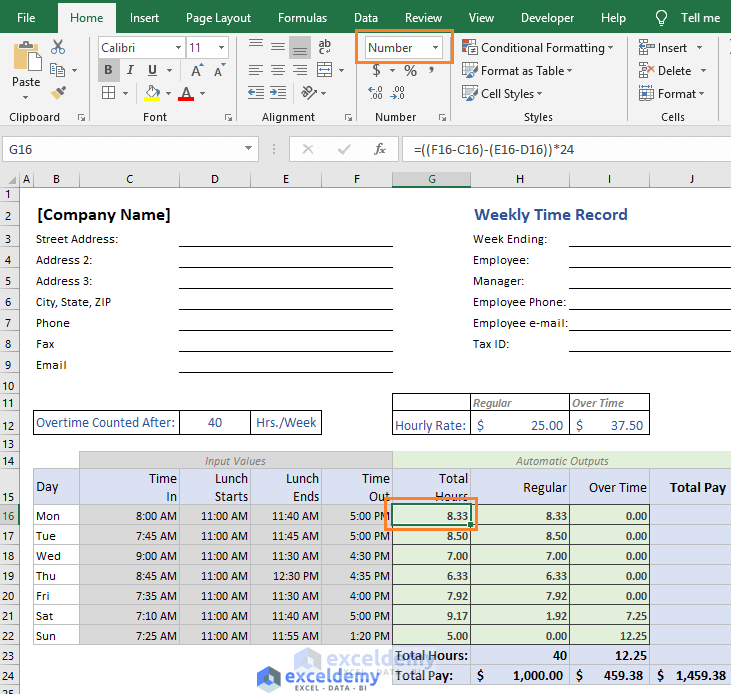2) Over Time Hours

Though the template shows the Regular Hours before the Over Time Hours, I actually calculated the Over Time Hours before the Regular Hours. This is the formula I have used in the cell I16 to calculate the Over Time Hours:

=IF(SUM(\$G\$16:G16)>work_hours_per_week,SUM(\$G\$16:G16)-work_hours_per_week,0)

Where, work_hours_per_week = D12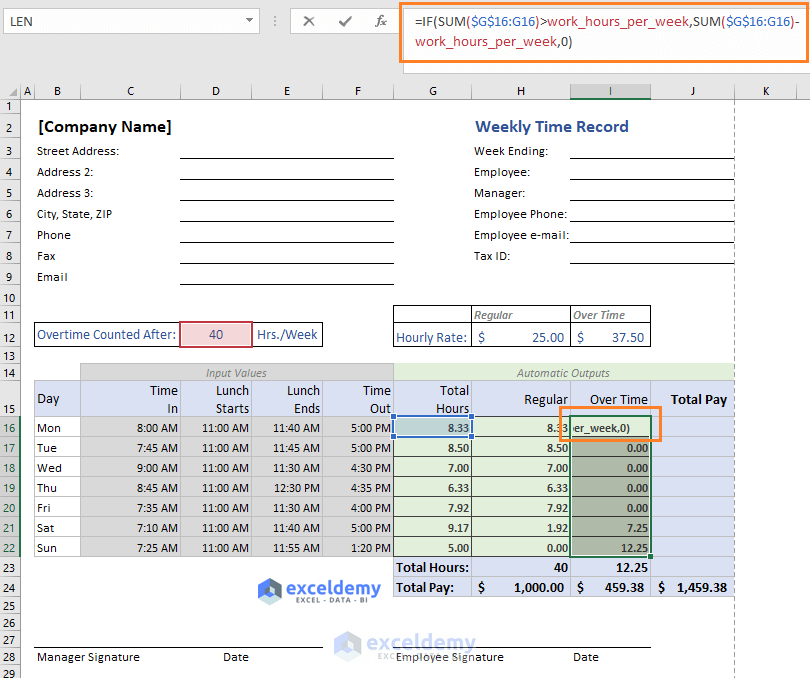How does this formula work?

• This formula has an expanding range. It is \$G\$16:G16. For the next cell (I17) in the column, this range will be: \$G\$16:G17. You see the range has expanded. This is why it is called expanding range.
• logical_test: IF function checks whether the sum of the expanding range has exceeded the value of work_hours_per_week.
• value_if_true: If the sum exceeds the value, the IF function returns this value: SUM(\$G\$16:G16)-work_hours_per_week.
• value_if_false: Otherwise the IF function returns value 0.

Let me check this formula for the cell I21: =IF(SUM(\$G\$16:G21)>work_hours_per_week,SUM(\$G\$16:G21)-work_hours_per_week,0)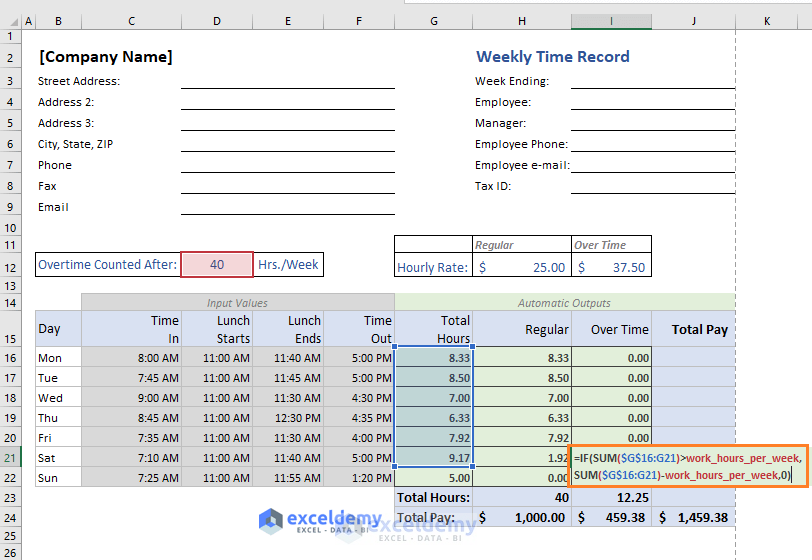• =IF(47.25>40,47.25-40,0); [I have replaced some parts of the formula with their values]
• =IF(TRUE,7.25,0)
• =7.25

So, the formula returns value 7.25.

3) Regular Hours

To calculate Regular Hours, I have used this formula in the cell H16: =MAX(G16-I16,0)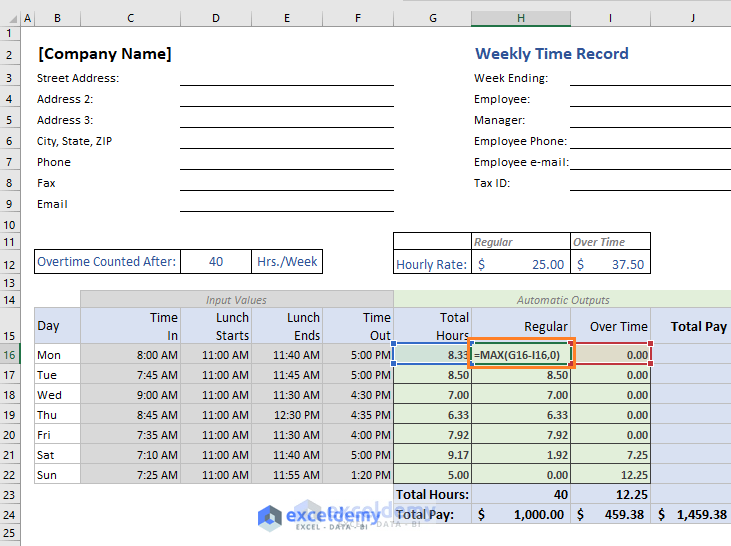This formula returns the maximum one between these two values: G16-I16 and 0.

4) Total Hours (Regular)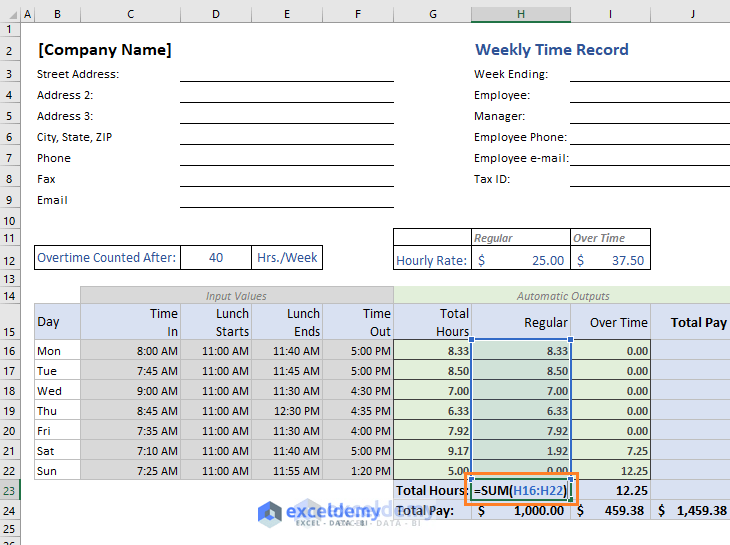5) Total Hours (Over Time)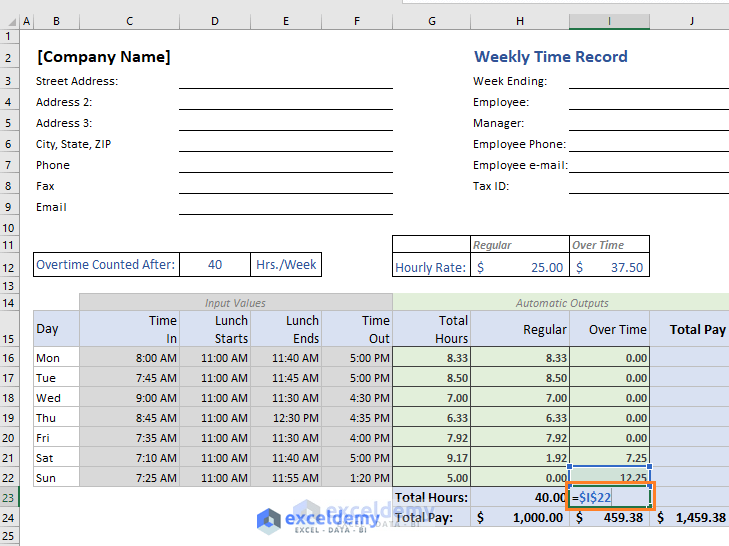Cell I22 actually holds our total Over Time hours. So, we just refer that cell in the cell I23.

6) Total Pay (Regular)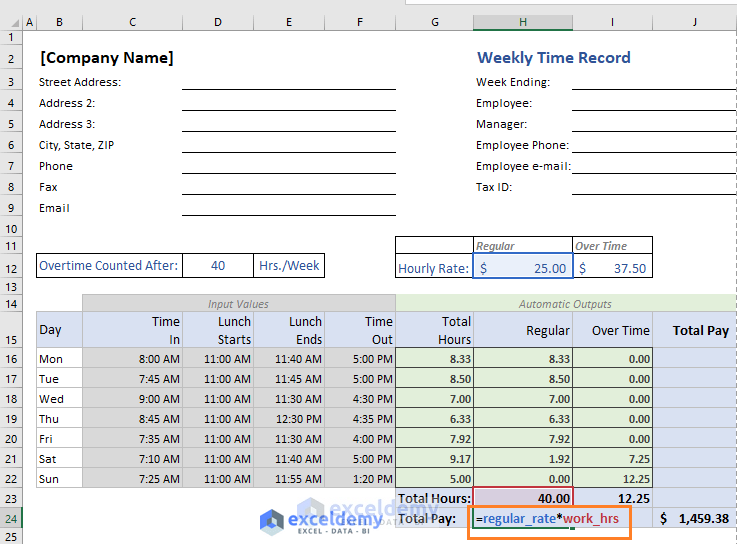Multiplication of the Regular Hourly Rate and total Regular Hours.

7) Total Pay (Over Time)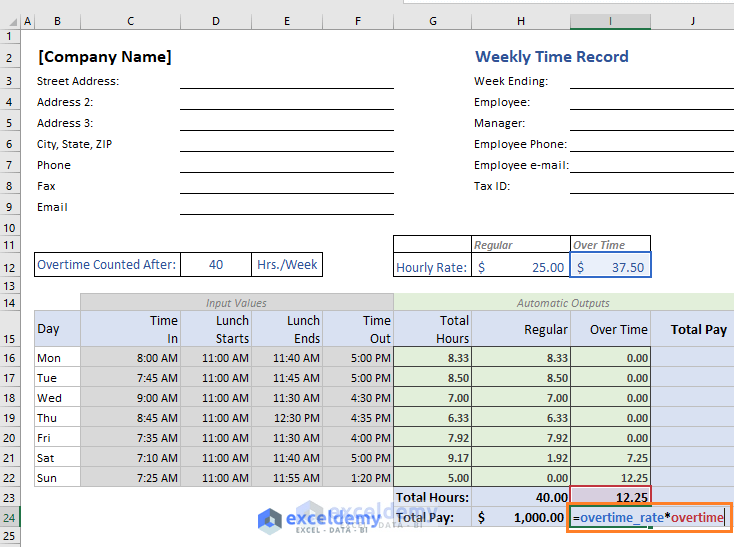Multiplication of the Over Time Rate and total Over Time Hours.

8) Grand Total Pay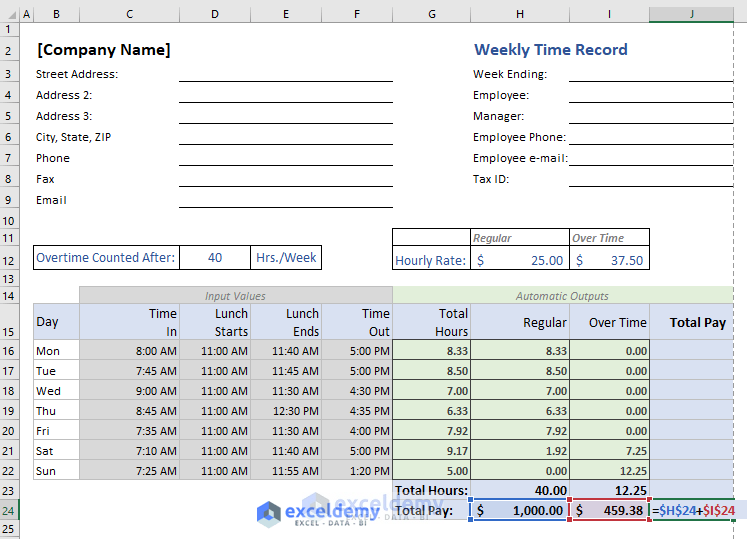Sum of Regular Pay and Over Time Pay.

## Conclusion

Whatever hourly rate your company provides and whatever working hours per week your company has adopted, this template will suit your company. Even if you did understand how the formulas work, you can modify and extend this template for fulfilling your specific criteria. So, these are the Excel formulas I use to calculate hours worked and overtime in a week.

Hello!
Welcome to my Excel blog! It took me some time to be a fan of Excel. But now I am a die-hard fan of MS Excel. I learn new ways of doing things with Excel and share here. Not only how to guide on Excel, but you will get also topics on Finance, Statistics, Data Analysis, and BI. Stay tuned!

1. ReplyR.K.Ghosh Jan 19, 2019 at 6:53 AM

Good. Send me learning module of Excel in my e-mail I’d: rajghosh116@gmail.com

• ReplyJan 19, 2019 at 8:00 PM

2. ReplyBrian Winkle Jan 21, 2019 at 8:08 PM

What if your company rounds the time? For example: When I clock in at 06:07 it calculates my time starting at 06:00 but if I were to clock in at 06:08, my start time is calculated at 06:15. By the way, Your emails are great. I highly enjoy them.

• ReplyJan 22, 2019 at 3:58 PM

I will check this issue and let you know. Glad to know that we can add some value via our emails.
Best regards
Kawser

3. ReplyJim Apr 11, 2019 at 10:23 PM

These formulas work pretty good if you work the 1st or 2nd shift. I work from 11:00pm – 7:30am with a 1/2 lunch period. When I enter these times, the formulas do not work. Any way to update this to work with these start and stop times?

4. ReplyMduduzi Oct 23, 2019 at 9:39 PM

Hi sir
Please send me practice data for advanced Excel so I can practice.

Thanks
Mdu

• ReplyOct 24, 2019 at 9:06 AM

You will also find the file in our article. The file is at the upper part of the article. You will find the file under this title: “Calculate Hours Worked and Overtime Excel Template”.
Best regards
Kawser Ahmed

5. Replyjoe frazier Mar 25, 2020 at 7:56 PM

In the overtime column, it is calculating the total overtime for the week. What if you wanted to just calculate the overtime hours for that day. For example in cell I22, instead of reading the total for the week (12.25) it just read the overtime for that day (5.0).

Thank You!!

6. ReplyChristina Corace Sep 16, 2020 at 2:42 AM

This was exactly what I was looking for! Is there a way for it to be multiple employees, in a table format verse just an individual log? Playing around with the formula now, seeing how I can enter more than one employee and the formula continues correctly

• ReplySep 16, 2020 at 11:14 PM

Thanks, Christina for your feedback. Glad to know that it helped you someway.